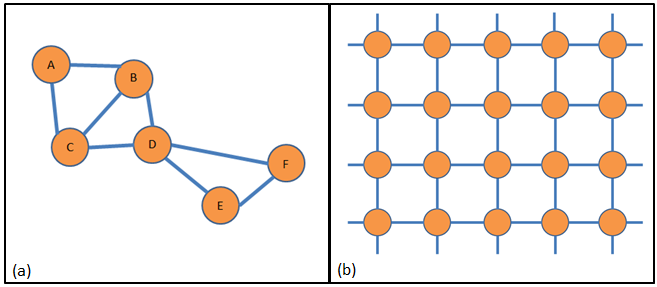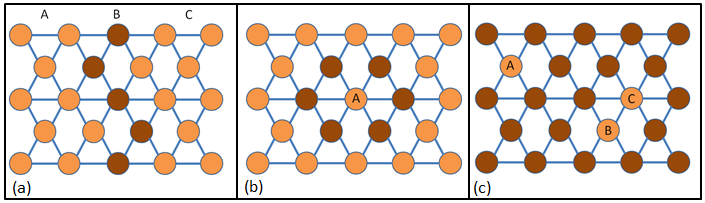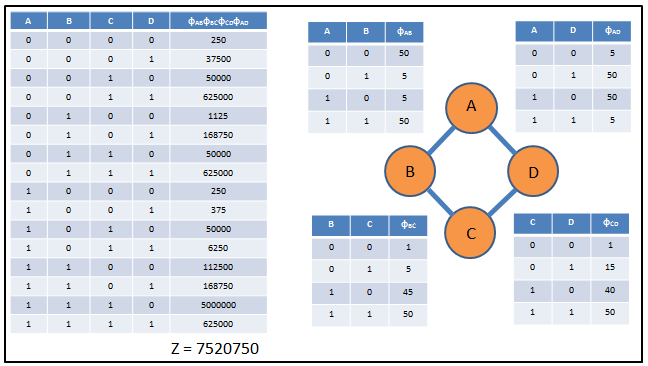# Course:CPSC522/Markov Networks

Jump to navigation Jump to search

## Markov Networks

A Markov network is an undirected graphical model that allows reasoning under uncertainty and which is often compared to Bayesian networks.

Principal Author: Jordon Johnson
Collaborators: Bahare

## Abstract

This page describes Markov networks as a graphical model for representing dependencies between random variables. It also describes the conditional independence properties of Markov networks and makes some comparisons between Markov and Bayesian representations of certain dependencies. Further, it gives an introduction to the representation of probabilities using factors over maximal cliques instead of conditional probability tables, and it works through a simple example.

### Builds on

Markov networks are a kind of graphical model that allow reasoning under uncertainty. They are often compared to Bayesian networks, with which they have both significant similarities and differences. The random variables represented by Markov networks may often be defined using predicate calculus.

### More general than

Markov networks can be considered more general than Bayesian networks in at least one respect: Markov networks are not required to be acyclic.

## Content

### Graphical Representation and ApplicationsFigure 1. Examples of Markov networks. (a) A simple Markov network with cyclical dependencies; for example, A is dependent on B, which is dependent on C, which is dependent on A. Note that the cycles can be traversed in either direction due to symmetry. (b) A portion of a large grid-like Markov network that could represent pixels in an image. Note that each node depends on its neighbors, with no hierarchy of dependence.

A Markov network is represented by an undirected graph $G=(V,E)$having the required Markov properties, where the nodes in $V$represent random variables, and the edges in $E$represent dependency relationships (see Figure 1). While this representation is similar to that of Bayesian networks, there are significant differences. The undirected edges in Markov networks allow symmetrical dependencies between variables, which are useful in fields such as image analysis, where the value of a given pixel depends on all the pixels around it, rather than having a hierarchy of pixels. In addition, Markov networks permit cycles, and so cyclical dependencies can be represented.

Markov networks are used in a variety of areas of artificial intelligence, including:

### Conditional Independence

#### Markov PropertiesFigure 2. The three Markov properties. (a) By the global property, the subgraphs A and C are conditionally independent given B. (b) By the local property, A is conditionally independent from the rest of the network given its neighbors. (c) By the pairwise property, A is conditionally independent from B and C given the rest of the network, but B and C are still dependent on each other.

It may be useful to summarize conditional independence in Markov networks as follows: any two parts of a Markov network are conditionally independent given evidence that "blocks" all paths between them. More formally, there are three key properties of Markov networks related to conditional independence:

• Global Markov property - Consider disjoint subsets $A$, $B$, and $C$of the Markov network. $A$and $C$are conditionally independent given $B$iff all paths connecting $A$and $C$pass through $B$.
• Undirected local Markov property - A node is conditionally independent from the rest of the network given its neighbors. Note that this means that the set of neighbors of a node constitute its Markov blanket (the smallest set of nodes that, when given as evidence, render the node conditionally independent from the rest of the network).
• Pairwise Markov property - Any two nodes in the Markov network are conditionally independent given the rest of the network if they are not neighbors.

It should be evident that the pairwise property follows from the local property, which in turn follows from the global property.

#### Markov vs. Bayesian NetworksFigure 3. Examples of instances where Markov networks cannot represent the conditional independence properties of Bayesian networks (and vice versa). (a) A and C are conditionally dependent given B in the Bayesian network but are conditionally independent in the similar Markov network. (b) (adapted from Koller and Friedman 2009, cited in Murphy 2012) In the Markov network, A and C are independent given B and D (and vice versa). The Bayesian version shown does not correctly represent these conditional independence relations.

Not all dependencies can be modeled with Markov networks; similarly, there are dependencies that can be modeled easily with Markov networks that cannot be modeled with Bayesian networks. Consider the examples shown in Figure 3.

Figure 3(a) shows a node with multiple parents, which is a fairly common structural feature in Bayesian networks. The parents are independent of each other in the absence of evidence, but they are conditionally dependent given the child. The corresponding Markov network represents both situations incorrectly; the outer nodes are dependent by transitivity in the absence of evidence but are conditionally independent given the middle node.

Figure 3(b) shows a cyclic dependence structure easily represented in a Markov network; opposing nodes are conditionally independent given their neighbors. While a Bayesian version of this captures the conditional independence of $A$and $C$given $B$and $D$, $B$and $D$are conditionally dependent given $C$.

If a probability distribution can be modeled perfectly (that is, representing all the dependence properties of the distribution without additional artefacts) by both Bayesian and Markov networks, then it is termed chordal.

### Probabilities and Factors

Since there are no parent-child relationships in Markov networks, we cannot assign a conditional probability table to each node and apply the chain rule as we do in Bayesian networks. Instead, we assign a factor to each maximal clique in the network. Note that these factors are not conditional probabilities; they are real-valued functions of the input variables that reflect the relative likelihood of the variables taking the specified values (see the worked example). These factors may be created manually by domain experts, or they may be learned from data. We can then use these factors to determine the probabilities of any desired assignment of values to the variables.

Let us consider $\mathbf {x}$, an assignment of values to the variables in a Markov network. Let us call $C$the set of maximal cliques in the network and assign a factor $\phi _{c}$to each clique $c\in C$. The probability $P(\mathbf {x} )$is then

$P(\mathbf {x} )={\frac {1}{Z}}\prod _{c\in C}{\phi _{c}(\mathbf {x} _{c})}$where the normalizing factor $Z$is

$Z=\sum _{\mathbf {x} }\prod _{c\in C}{\phi _{c}(\mathbf {x} _{c})}$For example, if we consider the Markov network shown in Figure 1(a), we can assign values as follows: $A=a$, $B=b$, and so on. Then we have

$P(a,b,c,d,e,f)={\frac {1}{Z}}\phi _{ABC}(a,b,c)\phi _{BCD}(b,c,d)\phi _{DEF}(d,e,f)$with $Z$being the sum over all possible assignments to the variables. Note that in the case of conditional probabilities, $Z$only includes assignments consistent with the evidence given.

#### A Simple Worked ExampleFigure 4. A simple Markov network with values given for the factors, products of factors, and normalization value $Z$.

Consider the simple Markov network from Figure 3(b), reproduced in Figure 4. Let $A$, $B$, $C$, and $D$be binary random variables representing four people's beliefs as to whether the Earth is round (1 for believes, 0 for does not believe). In this case, a fictitious domain expert has created the factors based on the following observations:

• Person A and person B tend to agree about things
• Person A and person D tend to disagree about things
• Person C tends to believe things are true, is more likely to be swayed by person D than by person B, and has no direct contact with person A
• Person B and person D have no direct contact

In this very simple network, the maximal cliques are simply pairs of nodes, and so each pair is assigned a factor with values given in Figure 4 (the factors have integer values in this example for the sake of simplicity). We obtain the products of factors for each possible assignment of values to the variables; for example, the product for ${A=0,B=1,C=0,D=1}$is simply

$\phi _{AB}(0,1)\phi _{BC}(1,0)\phi _{CD}(0,1)\phi _{AD}(0,1)=5*45*15*50=168750$We then sum these products to obtain $Z$, and can thence determine the probability of any assignment of the variables. Note that the most likely scenario in this example is that all but person D believe that the Earth is round (with probability ~0.66).

## Annotated Bibliography

Murphy, Kevin P. Machine learning: a probabilistic perspective. MIT press, 2012. Chapter 19: Undirected graphical models (Markov random fields)
"Markov random field." Wikipedia: The Free Encyclopedia. Wikimedia Foundation, Inc. 19 Dec. 2015. Web. 26 Jan. 2016.